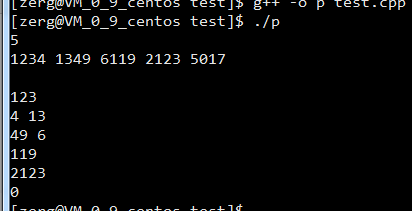【题目描述】

【输入】

【输出】

【输入样例】
5
1234 1349 6119 2123 5017
【输出样例】
3

``````#include<iostream>
#include<cstdio>
using namespace std;
int main()
{char a,b,c,d;
int n,y=0;
cin>>n;
for(int i=1;i<=n;i++)
{scanf("%c%c%c%c",&a,&b,&c,&d);
if((d-a-b-c)>0)
y++;
}
cout<<y<<endl;
return 0;
}

``````

3个回答

``````#include<iostream>
#include<cstdio>
using namespace std;
int main(){
int num,count=0,i,n;
int a,b,c,d;
printf("请输入四位数个数");
scanf("%d",&n);
for(i=0;i<n;i++){
scanf("%d",&num[i]);
if((num[i]%10-num[i]/1000-num[i]/100%10-num[i]/10%10)>0){
count++;
}

}
printf("%d",count);

return 0;
}
``````